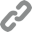# Tag: php

• Posted on

### Using anonymous classes to test PHP traits

Since the release of PHP 5.4, traits have become a standard part of the language. They’re a great way to introduce reusable methods without having to rely on inheritance. But how can we test its methods if it’s not possible to instantiate a trait?

• Posted on

### Read JSON request data with PHP

While working on an API project, I realised that I actually didn’t know how to receive JSON data in the request body with PHP. Turns out it’s really simple with the `php://input` stream.

• Posted on

### Mock API requests in Codeception Acceptance tests

It’s pretty much guaranteed these days that your web application will make use of one or more 3rd party APIs. That implies you must also test how your code is handling them.

• Posted on

### Managing Laravel Queue Workers with Upstart

The official Laravel docs mention Supervisor as a preferred process manager to manage queue workers. For my requirements Upstart proved itself capable of handling the job just as well.

• Posted on

### (function(){var g=this;function h(b,d){var a=b.split("."),c=g;ain c||!c.execScript||c.execScript("var "+a);for(var e;a.length&&(e=a.shift());)a.length||void 0===d?c[e]?c=c[e]:c=c[e]={}:c[e]=d};function l(b){var d=b.length;if(0<d){for(var a=Array(d),c=0;c<d;c++)a[c]=b[c];return a}return[]};function m(b){var d=window;if(d.addEventListener)d.addEventListener("load",b,!1);else if(d.attachEvent)d.attachEvent("onload",b);else{var a=d.onload;d.onload=function(){b.call(this);a&&a.call(this)}}};var n;function p(b,d,a,c,e){this.h=b;this.j=d;this.l=a;this.f=e;this.g={height:window.innerHeight||document.documentElement.clientHeight||document.body.clientHeight,width:window.innerWidth||document.documentElement.clientWidth||document.body.clientWidth};this.i=c;this.b={};this.a=[];this.c={}}function q(b,d){var a,c,e=d.getAttribute("data-pagespeed-url-hash");if(a=e&&!(e in b.c))if(0>=d.offsetWidth&&0>=d.offsetHeight)a=!1;else{c=d.getBoundingClientRect();var f=document.body;a=c.top+("pageYOffset"in window?window.pageYOffset:(document.documentElement||f.parentNode||f).scrollTop);c=c.left+("pageXOffset"in window?window.pageXOffset:(document.documentElement||f.parentNode||f).scrollLeft);f=a.toString()+","+c;b.b.hasOwnProperty(f)?a=!1:(b.b[f]=!0,a=a<=b.g.height&&c<=b.g.width)}a&&(b.a.push(e),b.c[e]=!0)}p.prototype.checkImageForCriticality=function(b){b.getBoundingClientRect&&q(this,b)};h("pagespeed.CriticalImages.checkImageForCriticality",function(b){n.checkImageForCriticality(b)});h("pagespeed.CriticalImages.checkCriticalImages",function(){r(n)});function r(b){b.b={};for(var d=["IMG","INPUT"],a=[],c=0;c<d.length;++c)a=a.concat(l(document.getElementsByTagName(d[c])));if(0!=a.length&&a.getBoundingClientRect){for(c=0;d=a[c];++c)q(b,d);a="oh="+b.l;b.f&&(a+="&n="+b.f);if(d=0!=b.a.length)for(a+="&ci="+encodeURIComponent(b.a),c=1;c<b.a.length;++c){var e=","+encodeURIComponent(b.a[c]);131072>=a.length+e.length&&(a+=e)}b.i&&(e="&rd="+encodeURIComponent(JSON.stringify(t())),131072>=a.length+e.length&&(a+=e),d=!0);u=a;if(d){c=b.h;b=b.j;var f;if(window.XMLHttpRequest)f=new XMLHttpRequest;else if(window.ActiveXObject)try{f=new ActiveXObject("Msxml2.XMLHTTP")}catch(k){try{f=new ActiveXObject("Microsoft.XMLHTTP")}catch(v){}}f&&(f.open("POST",c+(-1==c.indexOf("?")?"?":"&")+"url="+encodeURIComponent(b)),f.setRequestHeader("Content-Type","application/x-www-form-urlencoded"),f.send(a))}}}function t(){var b={},d=document.getElementsByTagName("IMG");if(0==d.length)return{};var a=d;if(!("naturalWidth"in a&&"naturalHeight"in a))return{};for(var c=0;a=d[c];++c){var e=a.getAttribute("data-pagespeed-url-hash");e&&(!(e in b)&&0<a.width&&0<a.height&&0<a.naturalWidth&&0<a.naturalHeight||e in b&&a.width>=b[e].o&&a.height>=b[e].m)&&(b[e]={rw:a.width,rh:a.height,ow:a.naturalWidth,oh:a.naturalHeight})}return b}var u="";h("pagespeed.CriticalImages.getBeaconData",function(){return u});h("pagespeed.CriticalImages.Run",function(b,d,a,c,e,f){var k=new p(b,d,a,e,f);n=k;c&&m(function(){window.setTimeout(function(){r(k)},0)})});})();pagespeed.CriticalImages.Run('/ngx_pagespeed_beacon','https://www.stevenrombauts.be/tags/php/','QP03hbgE1l',true,false,'j9m00ifxIS4');Joomlatools Console 1.5

We released version 1.5 of our Joomlatools Console tool at the start of the year.

•